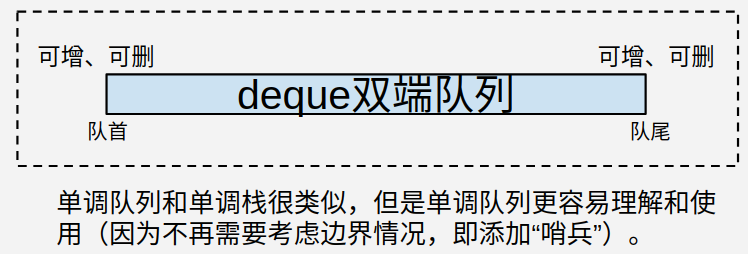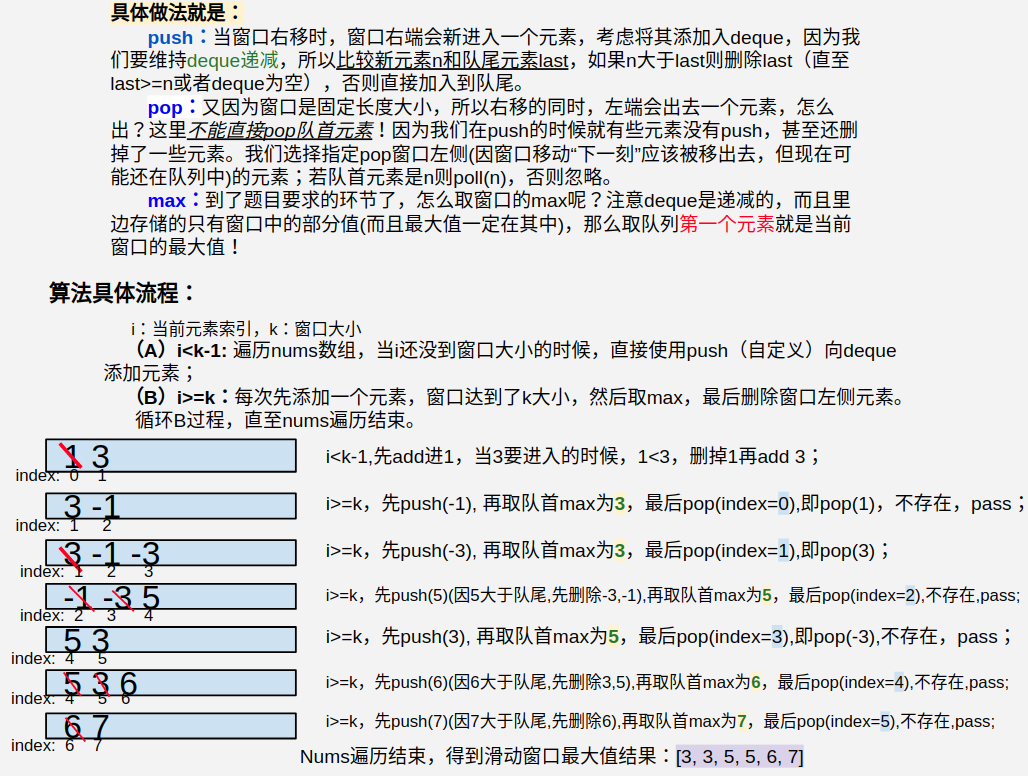• 给你一个zheng’s数组 nums，有一个大小为 k 的滑动窗口从数组的最左侧移动到数组的最右侧。你只可以看到在滑动窗口内的 k 个数字。滑动窗口每次只向右移动一位。 返回滑动窗口中的最大值。 示例 1： 输入：nums...

给你一个zheng’s数组 nums，有一个大小为 k 的滑动窗口从数组的最左侧移动到数组的最右侧。你只可以看到在滑动窗口内的 k 个数字。滑动窗口每次只向右移动一位。

返回滑动窗口中的最大值。

示例 1： 输入：nums = [1,3,-1,-3,5,3,6,7], k = 3 输出：[3,3,5,5,6,7] 解释： 滑动窗口的位置 最大值

[1 3 -1] -3 5 3 6 7 3 1 [3 -1 -3] 5 3 6 7 3 1 3 [-1 -3 5] 3 6 7 5 1 3 -1 [-3 5 3] 6 7 5 1 3 -1 -3 [5 3 6] 7 6 1 3 -1 -3 5 [3 6 7] 7

示例 2： 输入：nums = , k = 1 输出：

示例 3： 输入：nums = [1,-1], k = 1 输出：[1,-1]

示例 4： 输入：nums = [9,11], k = 2 输出：

示例 5： 输入：nums = [4,-2], k = 2 输出：

class Solution {
public int[] maxSlidingWindow(int[] nums, int k) {
// 判断
if(nums==null || nums.length<2 || k==1) {
return nums;
}
// 维护一个队头元素始终是队列最大
// 结果数组
int[] res = new int[nums.length-k+1];
// 遍历
for(int i=0;i<nums.length;i++) {

// 放入的元素已经够窗口的值了 删除过期值
while(!queue.isEmpty()&&queue.peekFirst()<=i-k) {
queue.pollFirst();
}
// 判断放入的值是否大于
while(!queue.isEmpty()&&nums[queue.peekLast()]<=nums[i]) {
queue.pollLast();
}
// 放入
// 判断窗口长度是否大于等于k个的时候才开始计算
if(i-k+1>=0) {
res[i-k+1] = nums[queue.peekFirst()];
}
}
return res;
}
}

展开全文双向队列
• 1. 例题 Leetcode 239题目： 输入: nums = [1,3,-1,-3,5,3,6,7], 和 k = 3 ...滑动窗口的位置 最大值 --------------- ----- [1 3 -1] -3 5 3 6 7 3 1 [3 -1 -3] 5 3 6 7 3 1 3 [-1 -3 5] 3 6 7...
1. 例题
Leetcode 239题目：
输入: nums = [1,3,-1,-3,5,3,6,7], 和 k = 3
输出: [3,3,5,5,6,7]
解释:

滑动窗口的位置                最大值
---------------               -----
[1  3  -1] -3  5  3  6  7       3
1 [3  -1  -3] 5  3  6  7       3
1  3 [-1  -3  5] 3  6  7       5
1  3  -1 [-3  5  3] 6  7       5
1  3  -1  -3 [5  3  6] 7       6
1  3  -1  -3  5 [3  6  7]      7
2. 单调队列（双端队列）
核心思想是维持deque/双端队列中的元素保持递增or递减。
使用该数据结构的优点是deque在队列两端都可以添加、删除元素，这里借助了它其中4种常数时间复杂度的操作(java)：offerLast(n)、getFirst()、pollFirst()、pollLast()。单调栈介绍详见：https://blog.csdn.net/LutherK/article/details/107023543
另外：单调队列还被应用于“多重背包问题”的优化解法。
3. 图解
思路：
在一堆数字中，已知最值，如果给这堆数添加一个数，那么比较一下就可以很快算出最值；但如果减少一个数，就不一定能很快得到最值了，而要遍历所有数重新找最值。
首先考虑，要在O(N)的时间内解决此问题，我们需要3个O(1)的操作帮助我们完成，分别是：
push(n)：在队列末尾添加元素n；
max：取当前队列/窗口中的最大值；
pop(n)：r若队首元素是n，则删除n；
在Java中的实现如下：
// 用于"单调队列"的成员内部类
private static class MonotonicQueue {
Deque<Integer> deque = new ArrayDeque<>();
void push(int n){
while(!deque.isEmpty() && deque.getLast()<n)
deque.pollLast();
deque.offerLast(n);
}
//
int max(){
return deque.getFirst();
}
//
void pop(int n){
if(!deque.isEmpty() && deque.getFirst()==n)
deque.pollFirst();
}
}4. 完整Java代码
    // (2) 双端队列（单调队列）
public static int[] maxSlidingWindow2(int[] nums, int k) {
int n = nums.length;
MonotonicQueue window = new MonotonicQueue();
int[] res = new int[n-k+1];
for(int i=0;i<n;i++){
if(i<k-1)
window.push(nums[i]);
else {
window.push(nums[i]);
res[i-k+1] = window.max();
window.pop(nums[i-k+1]);
}
}
return res;
}

// 用于"单调队列"的成员内部类
private static class MonotonicQueue {
Deque<Integer> deque = new ArrayDeque<>();
void push(int n){
while(!deque.isEmpty() && deque.getLast()<n)
deque.pollLast();
deque.offerLast(n);
}
//
int max(){
return deque.getFirst();
}
//
void pop(int n){
if(!deque.isEmpty() && deque.getFirst()==n)
deque.pollFirst();
}
}
5. 另一种思路
双向队列
复杂度
时间 O(N) 空间 O(K)
思路
我们用双向队列可以在O(N)时间内解决这题。当我们遇到新的数时，将新的数和双向队列的末尾比较，如果末尾比新数小，则把末尾扔掉，直到该队列的末尾比新数大或者队列为空的时候才住手。这样，我们可以保证队列里的元素是从头到尾降序的，由于队列里只有窗口内的数，所以他们其实就是窗口内第一大，第二大，第三大...的数。保持队列里只有窗口内数的方法和上个解法一样，也是每来一个新的把窗口最左边的扔掉，然后把新的加进去。然而由于我们在加新数的时候，已经把很多没用的数给扔了，这样队列头部的数并不一定是窗口最左边的数。这里的技巧是，我们队列中存的是那个数在原数组中的下标，这样我们既可以直到这个数的值，也可以知道该数是不是窗口最左边的数。这里为什么时间复杂度是O(N)呢？因为每个数只可能被操作最多两次，一次是加入队列的时候，一次是因为有别的更大数在后面，所以被扔掉，或者因为出了窗口而被扔掉。
public class Solution {
public int[] maxSlidingWindow(int[] nums, int k) {
if(nums == null || nums.length == 0) return new int;
int[] res = new int[nums.length + 1 - k];
for(int i = 0; i < nums.length; i++){
// 每当新数进来时，如果发现队列头部的数的下标，是窗口最左边数的下标，则扔掉
if(!deque.isEmpty() && deque.peekFirst() == i - k) deque.poll();
// 把队列尾部所有比新数小的都扔掉，保证队列是降序的
while(!deque.isEmpty() && nums[deque.peekLast()] < nums[i]) deque.removeLast();
// 加入新数
deque.offerLast(i);
// 队列头部就是该窗口内第一大的
if((i + 1) >= k) res[i + 1 - k] = nums[deque.peek()];
}
return res;
}
}

补充：
此题还有另外一种解法，时间复杂度也是O(N)，可以说是DP，也可是看做“同步双向遍历”。
代码：

 // （1）动态规划
// left[i] 表示从窗口开始到下标i的最大值
// right[j]  表示从窗口末尾到索引j的最大值
public static int[] maxSlidingWindow(int[] nums, int k) {
int n = nums.length;
if (n * k == 0) return new int;
if (k == 1) return nums;

int[] left = new int[n];
left = nums;
int[] right = new int[n];
for (int i = 1; i < n; i++) {
if (i%k==0) left[i] = nums[i];
else
left[i] = Math.max(left[i-1],nums[i]);
int j = n-1-i;
if((j+1)%k==0)
right[j] = nums[j];
else
right[j] = Math.max(right[j+1],nums[j]);
}
int[] res = new int[n-k+1];
for (int i = 0; i <= n - k; i++) {
res[i] = Math.max(right[i],left[i+k-1]);
}
return res;
}


展开全文• 给定一个数组nums，有一个大小为k的滑动窗口从数组的最左侧移动到数组的最右侧。你只可以看到在滑动窗口k内的数字。滑动窗口每次只向右移动一位。 返回滑动窗口最大值。 示例: 输入: nums = [1,3,-1,-3,5,3,6,7]...
给定一个数组 nums，有一个大小为 k 的滑动窗口从数组的最左侧移动到数组的最右侧。你只可以看到在滑动窗口 k 内的数字。滑动窗口每次只向右移动一位。
返回滑动窗口最大值。
示例:
输入: nums = [1,3,-1,-3,5,3,6,7], 和 k = 3
输出: [3,3,5,5,6,7]
解释:

滑动窗口的位置                最大值
---------------               -----
[1  3  -1] -3  5  3  6  7       3
1 [3  -1  -3] 5  3  6  7       3
1  3 [-1  -3  5] 3  6  7       5
1  3  -1 [-3  5  3] 6  7       5
1  3  -1  -3 [5  3  6] 7       6
1  3  -1  -3  5 [3  6  7]      7
注意：
你可以假设 k 总是有效的，1 ≤ k ≤ 输入数组的大小，且输入数组不为空。
进阶：
你能在线性时间复杂度内解决此题吗？

class Solution
{
public:
vector<int> maxSlidingWindow(vector<int> &nums, int k)
{
deque<int> window;
vector<int> ans;
for (int i = 0; i < nums.size(); i++)
{
if(i >= k && i - k + 1 > window.front())
window.pop_front();
while(!window.empty() && nums[window.back()] < nums[i])
{
window.pop_back();
}
window.push_back(i);
if(i >= k - 1)
{
ans.push_back(nums[window.front()]);
}
}
return ans;
}
};

展开全文• 239. 滑动窗口最大值 Sliding Window Maximum LeetCodeCN 第239题链接 第一种方法：用优先队列：大顶堆 第二种方法：因为窗口大小固定，只需要一个双端队列即可 class Solution: def maxSlidingWindow(self, nums: ...
有关栈、堆、队列的LeetCode做题笔记，Python实现
239. 滑动窗口最大值 Sliding Window Maximum
LeetCodeCN 第239题链接
第一种方法：用优先队列：大顶堆
第二种方法：因为窗口大小固定，只需要一个双端队列即可
class Solution:
def maxSlidingWindow(self, nums: List[int], k: int) -> List[int]:
if not nums:
return []
window, res = [], []
for i, x in enumerate(nums):
if i >= k and window <= i - k:
window.pop(0)
while window and nums[window[-1]] <= x:
window.pop()
window.append(i)
if i >= k - 1:
res.append(nums[window])
return res

展开全文Python
• 单调队列=滑动窗口内的最值 84. 柱状图中最大的矩形 思路： 如何枚举所有的情况？枚举所有柱形的上边界，作为整个矩形的上边界 然后找出左右边界，找出左边离它最近，比它小且最高的矩形；找出右边离它最近，比它小...
• 单调双端队列（使用单调的队列）的意义在于，可以排除那些老的，对数组没有用的数。从左到右代表时间顺序，右边添加，左边删除。 from collections import deque class Solution: def longestSubarray(self, nums...
• 1. 单调队列（双端队列） 核心思想是维持deque/双端队列中的元素保持递增or递减。...单调介绍详见：https://blog.csdn.net/LutherK/article/details/107023543 另外：单调队列还被应用于“多重背包...数据结构 算法 java
• 给定一个数组 nums，有一个大小为k的滑动窗口从数组的最左侧移动到数组的最右侧。你只可以看到在滑动窗口内的 k个数字。滑动窗口每次只向右移动一位。 返回滑动窗口中的最大值。 进阶： 你能在线性时间复杂度内...
• 有关子串、子数组问题都可以考虑滑动窗口 我自己写的窗口滑动的题的大致模板是这样的： for i ,c in enumerate(s): if 不满足条件： 进行下一个循环 看是否需要更新什么值
• 如何配合窗口移动产生的移除元素代码实现： Leetcode239 1.问题描述 2.解决方案 解法一：暴力(可行，太慢) 解法二：优先队列(不可行) 解法三：维护一部分元素的单调队列(可行) 前导分析： 引出问题： 1,...数据结构 python
• 给你一个整数数组 nums，有一个大小为 k 的滑动窗口从数组的最左侧移动到数组的最右侧。你只可以看到在滑动窗口内的 k 个数字。滑动窗口每次只向右移动一位。 返回滑动窗口中的最大值。 Example 1: 输入：nums = [1...算法 python 数据结构
• LeetCode 滑动窗口（Sliding Window）类问题总结 滑动窗口算法框架 //滑动窗口基本框架 //不要记框架，重点是理解滑动窗口的思想 int slidingWindowTemplate(String a, ...) { // 输入参数有效性判断 if (...) { ...算法
• 给你一个整数数组 nums，有一个大小为 k 的滑动窗口从数组的最左侧移动到数组的最右侧。你只可以看到在滑动窗口内的 k 个数字。滑动窗口每次只向右移动一位。 返回滑动窗口中的最大值。 示例： 输入：nums = [1,3,-...队列
• 给你一个整数数组 nums，有一个大小为 k 的滑动窗口从数组的最左侧移动到数组的最右侧。你只可以看到在滑动窗口内的 k 个数字。滑动窗口每次只向右移动一位。 返回滑动窗口中的最大值。 解答： 方法一：单调递减...算法
• 滑动窗口最大值 题目 给定一个数组 nums，有一个大小为 k 的滑动窗口从数组的最左侧移动到数组的最右侧。你只可以看到在滑动窗口内的 k 个数字。滑动窗口每次只向右移动一位。 返回滑动窗口中的最大值。 进阶： 你...
• 给定一个数组 nums，有一个大小为 k 的滑动窗口从数组的最左侧移动到数组的最右侧。你只可以看到在滑动窗口内的 k 个数字。滑动窗口每次只向右移动一位。 返回滑动窗口中的最大值。 示例: 输入: nums = [1,3,-1,-3,...双端队列
• 239. 滑动窗口最大值 给定一个数组 nums，有一个大小为 k 的滑动窗口从数组的最左侧移动到数组的最右侧。你只可以看到在滑动窗口内的 k 个数字。滑动窗口每次只向右移动一位。 返回滑动窗口中的最大值。 进阶： 你能...队列 数据结构 算法 python
• 来源：力扣（LeetCode） ...给定一个数组 nums 和滑动窗口的大小 k，请找出所有滑动窗口里的最大值。 示例 输入: nums = [1,3,-1,-3,5,3,6,7], 和 k = 3 输出: [3,3,5,5,6,7] 解释: 滑动窗口的位置java 数据结构
• 1. 运用滑动窗口模板解题, 具体可以看滑动窗口总结这篇. 2. 但是我们需要注意每次类似维护一个单调递减,这样front就是最大值,然后移除之后,下一个就是下一个窗口的最大值. 3. 遇到大于back值的,就循环将最大值...
• 给你一个整数数组 nums，有一个大小为 k 的滑动窗口从数组的最左侧移动到数组的最右侧。你只可以看到在滑动窗口内的 k 个数字。滑动窗口每次只向右移动一位。 返回滑动窗口中的最大值。 示例 1： 输入：nums = [1,3,...数据结构 算法 stack
•数据结构 算法
• 给你一个整数数组 nums，有一个大小为 k 的滑动窗口从数组的最左侧移动到数组的最右侧。你只可以看到在滑动窗口内的 k 个数字。滑动窗口每次只向右移动一位。 返回滑动窗口中的最大值。 示例 1： 输入：nums = [1,3...
• 239. 滑动窗口最大值 题意: 给你一个整数数组 nums，有一个大小为 k 的滑动窗口从数组的最左侧移动到数组的最右侧。你只可以看到在滑动窗口内的 k 个数字。滑动窗口每次只向右移动一位。 返回滑动窗口中的最大值。 ...队列 数据结构 算法 c++...